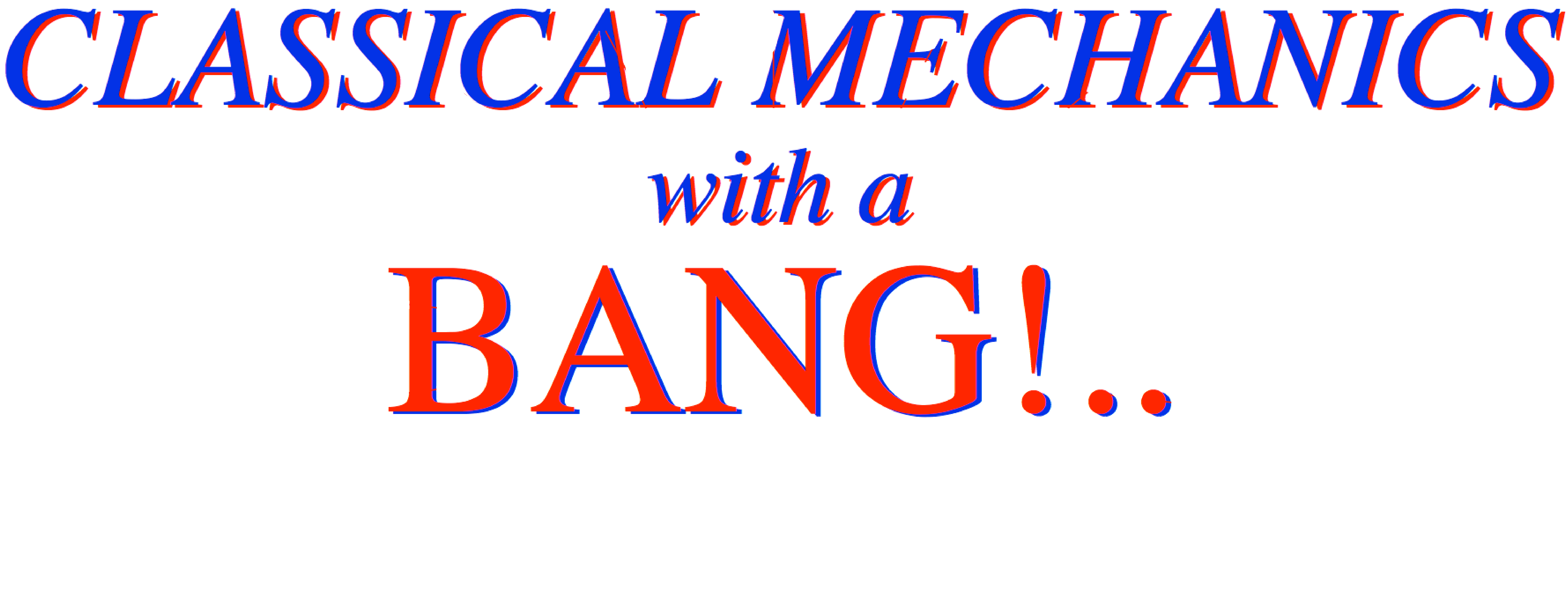Text Topics for Units 1-8
Introduction to geometry and algebra of mechanics fundamentals by plane geometry
Review of fields and their vector analysis by geometry and complex variables
Superball missile and neutron starlet dynamics. Coupled oscillator and rotational motion
Introduction to Hamiltonian, Estrangian, and Lagrangian contact mechanics of Action
Chapter 1.  Collision velocity change and slope geometry ......... Page 23
Chapter 2.  Velocity and momentum ......... Page 37
Chapter 3.  Velocity and energy ......... Page 45
Chapter 4.  Dynamics and geometry of successive collisions ......... Page 55
Chapter 5.  Multiple collisions and operator analysis ......... Page 69
Chapter 6.  Introducing Force, Potential Energy, and Action ......... Page 79
Chapter 7.  Interaction Forces and Potentials in Collisions ......... Page 91
Chapter 8.  N-Body Collisions: Two’s company but three’s a crowd ......... Page 105
Chapter 9.  Geometry and physics of common potential fields ......... Page 119
Chapter 10.  Calculus of exponentials, logarithms, and complex fields ......... Page 135
Chapter 11.  Oscillation, Rotation, and Angular Momentum ......... Page 167
Chapter 12.  Velocity vs momentum functions: Lagrange vs Hamilton ......... Page 135
Appendix 1A.  Vector product geometry and Levi-Civita εijk ......... Page 1A1

Riemann-Christoffel covariant tensor equations of motion and differential geometry
Effective potentials and geometry of constraints and Lagrange multipliers

Lorentz resonance response and Fourier analysis
Normal modes and U(2) Euler angle geometry of pair resonance
Fourier and symmetry analysis of wave dispersion and parametric resonance

Coulomb and central-force orbits and trajectory envelopes
Rutherford orbit geometry
U(2) and R(4) geometry of oscillator and Coulomb dynamics
Rutherford, Stark, Zeeman, and 2-center orbits

2-particle scattering.
Angular rotation and momentum of gyros, tops, spacecraft, and molecules
Euler-angle geometry and rotational energy surface analysis of soft rotors

Calculus of variation and Hamilton-Jacobi equations
Geometry of contact transformations
Semiclassical action quantization by Davis-Heller phase color addition

Optical dispersion derivation of relativistic quantum mechanics
Chaotic motion. Optimal control theory

Defining Constants
Current Best Estimates (IAU 2009)
Constants from IAU WG on Cartographic Coordinates and Rotational Elements (2007)
Other Constants